# 1. Introduction: 20% of the leetcode problems.

At the beginning when we want to recursively solve a problem on LeetCode, what do you come up in your mind? It is terminologies like Divide and Conquer ∪ Dynamic Programming ∪ Backtracking(searching with BFS or DFS).

Divide and conquer partitions the problems into disjoint subproblems and solves the problems recursively, and then combine the solutions to solve the original problem. Dynamic programming is an optimized Divide and conquer, which solves each sub-problem only once and save its answer in a table. There is no recursion. The key in dynamic programming is memoization. Dynamic programming applies when the subproblems overlap — that is, when subproblems share subproblems.

For me, it is a lot easier to use two recursion functions to show the difference of each other.

• Divide and Conquer: T(n)=aT(n/b)+f(n), (for problems that each element can be added to the result or not, usually half and half, normally with lower complexity than brute force, and no need of memoization)
• Dynamic Programming: T(n) = T(n-1)+T(n-2)+…+f(n). (for problems that need to split,cutting 2^n, we can use memoization and iterative method). Two ways to implement dp, bottom-to-top and top-to-bottom.

However, Backtracking searching which usually we see problems in the form of combination and permutation, it is just a way to enumerate all the solutions that is within the constraint condition.

Note: It is very important to differentiate these two subcategories, lessons learned from one Facebook interview.

# 2. Divide and Conquer

Normal steps:

1. Draw examples, then we use a recursion-tree to “divide” the problems into subproblems.

2. Solve the “base Case”, whose size usually is 1.

3. Merge the results from the subproblems.

4. Observe for possible optimization, repeated subprolems we can use “memorization”

4. Use coding language to streamline the process

5. check more edge cases, and the indexes, e.g. low, high, mid

6. Analyze the time and space complexity

Warning: Divide and Conquer can be used to solve the problems. However, it cant get to Best Conveivable Runtime (BCR). For array it is O(n). Therefore, when we brain storming to find better solution, think about other algorithms. For array/string: Sliding window, two pointers with O(n).

# 3. Dynamic Programming

For dynamic programming, it is very important to come up with the recursion function. Commonly we have:

• T(n)=T(n-1)+T(n-2)+…+T(n-k). k in [1,n-1]
• T(n)=max(cost+T(left1), T(left2)), for optimization problems, choose or not choose.
• T(n)=T(0,i-1)optT(i+1,n) (i=[1,n]), including a for loop to set up splitting position i. opt is used to connect the return result of each side, two states are split or not split yet.

Two approaches :

• bottom-up (iterative): we build the solution for one case off of the previous case (or multiple cases), for fibonacci(3)=fibonacci(2)+fibonacci(1), then we go to fibonacci(4). Time complexity is O(n), the same with top-down with memorization (DP+Memo).

Normally: 1. base cases() and special cases 2. update function, 3. goal, what is the return. And it is harder to write compared with top-down recursive solutions

• Top-down (recursive): in this case, we think about how to divide the problem for the case N into subproblems. Normally we use recursive and memo.

Note: recursive algorithms can be very space inefficient. Each recursive call adds a new layer to the stack, which means that if your algorithm recurses to a depth of n, it uses at least O(n) memory.

For this reason, it’s often better to implement a recursive algorithm iteratively. All recursive algorithms can be implemented iteratively, although sometimes the code to do so is much more complex. Before diving into the recursive code, ask yourself how hard it would be to implement it iteratively, and discuss the tradeoffs with your interviewers.

# 4. Backtracking+(BFS or DFS)

Please check my other post for the introduction of backtracking.

Now, finally understood the difference of these three. Let use see how they are applied to different types of questions.

# 5. Examples

## 5.1 Standard Divide and Conquer: T(n)=aT(n/b)+f(n)

T(n) = op(T(left),T(right)), where op usually starts after we get the return result from T(left), T(right). op means the operation we need to “merge” the result, if the cost for this merge operation is f(n). For this type of divide and conquer, most time, it has smaller running time complexity compared with the brute-force.

Note: get more examples to testify if this actually work.

The example is merge sort (T(n) = 2T(n/2)+n for merge the return), maximum subarray,

53. Maximum Subarray

Find the contiguous subarray within an array (containing at least one number) which has the largest sum.

For example, given the array `[-2,1,-3,4,-1,2,1,-5,4]`,
the contiguous subarray `[4,-1,2,1]` has the largest sum = `6`.

1. divide and conquer solution: T(n) = max(T(left),T(right), T(cross)), max is for merging and the T(cross) is for the case that the potential subarray across the mid point. For the complexity, T(n)=2T(n/2)+n, if we use the master method, it would give us O(nlgn).
`def maxSubArray(self, nums):        """        :type nums: List[int]        :rtype: int        """        def getCrossMax(low,mid,high):            left_sum,right_sum =0,0            left_max,  right_max = -maxint, -maxint            left_i,right_j=-1,-1            for i in xrange(mid,low-1,-1): #[)                left_sum+=nums[i]                if left_sum>left_max:                    left_max= left_sum                    left_i = i            for j in xrange(mid+1,high+1):                right_sum+=nums[j]                if right_sum>right_max:                    right_max= right_sum                    right_j = j            return (left_i,right_j,left_max+right_max)                def maxSubarray(low,high):            if low==high:                return (low,high, nums[low])            mid = (low+high)//2            rslt=[]            #left_low, left_high, left_sum = maxSubarray(low,mid) #[low,mid]            rslt.append(maxSubarray(low,mid)) #[low,mid]            #right_low,right_high,right_sum = maxSubarray(mid+1,high)#[mid+1,high]            rslt.append(maxSubarray(mid+1,high))            #cross_low,cross_high,cross_sum = getCrossMax(low, mid, high)            rslt.append(getCrossMax(low, mid, high))            return max(rslt, key=lambda x: x)        return maxSubarray(0,len(nums)-1)`
1. convert this problem to best time to buy and sell stock problem.[0, -2, -1, -4, 0, -1, 1, 2, -3, 1], => O(n)
2. use prefix_sum, the difference is we set prefix_sum to 0 when it is smaller than 0, O(n)
`from   sys import maxintclass Solution(object):        def maxSubArray(self, nums):        """        :type nums: List[int]        :rtype: int        """        max_so_far = -maxint - 1        prefix_sum= 0        for i in range(0, len(nums)):            prefix_sum+= nums[i]            if (max_so_far < prefix_sum):                max_so_far = prefix_sum             if prefix_sum< 0:                prefix_sum= 0          return max_so_far`

50. Pow(x, n)

T(n)= T(n/2)+O(1), the complexity is the same as the binary search, O(logn).

`def myPow(self, x, n):        """        :type x: float        :type n: int        :rtype: float        """        if n==0:            return 1        if n<0:            n=-n            x=1.0/x        def helper(n):            if n==1:                return x                        h = n//2            r = n-h            value = helper(h) #T(n/2), then we have O(1)            if r==h:                                return value*value            else: #r is going to be 1 bigger                return value*value*x        return helper(n)`

198. House Robber

If we use brute force is O(2^n). Use divide and conquer, here because we use half and half, so memo is useless.

`def rob(self, nums):        """        :type nums: List[int]        :rtype: int        """        memo=[[-1 for _ in range(len(nums))] for _ in range(len(nums))]                def dp(l,r):            nonlocal memo            if l==r:                return nums[l]            if l>r:                return 0            if l<r:                if memo[l][r]==-1:                    mid=l+(r-l)//2                    value = nums[mid] +dp(l,mid-2)+dp(mid+2,r)#take this value                    not_value =dp(l,mid-1)+dp(mid+1,r) #not take                    memo[l][r]=max(value, not_value)                return memo[l][r]        return dp(0,len(nums)-1)`

321. Create Maximum Number

Given two arrays of length `m` and `n` with digits `0-9` representing two numbers. Create the maximum number of length `k <= m + n` from digits of the two. The relative order of the digits from the same array must be preserved. Return an array of the `k` digits. You should try to optimize your time and space complexity.

Example 1:

nums1 = `[3, 4, 6, 5]`
nums2 = `[9, 1, 2, 5, 8, 3]`
k = `5`
return `[9, 8, 6, 5, 3]`

Example 2:

nums1 = `[6, 7]`
nums2 = `[6, 0, 4]`
k = `5`
return `[6, 7, 6, 0, 4]`

Example 3:

nums1 = `[3, 9]`
nums2 = `[8, 9]`
k = `3`
return `[9, 8, 9]`

Solution: First use DP + memo: LTE, 70/120 passed. We should use greedy and solve each array to find i,j, i+j = k, then we combine them.

`def maxNumber(self, nums1, nums2, k):        """        :type nums1: List[int]        :type nums2: List[int]        :type k: int        :rtype: List[int]        """        '''i, j T(i,j,k)= max(nums[i]*10^k-1+T(i+1,j,k-1), j, T(i+1,j+1,k)), for the third iterm, the restriction is we need the left       elements>=k'''        n1=len(nums1)        n2=len(nums2)        memo=[[[None for k in range(k+1)] for col in range(n2+1) ] for row in range(n1+1)]        def dp(i,j,k):            if k==0:                return 0            if memo[i][j][k] is None:                max1,max2,max3=-1,-1,-1                if i<len(nums1):                    tmp =dp(i+1, j, k-1) #take i                    tmp2 = dp(i+1,j,k) #neglect i in nums1                    if tmp!=-1:                        max1 = nums1[i]*(10**(k-1))+tmp                    max1=max(max1,tmp2)                if j<len(nums2):                    tmp = dp(i, j+1, k-1) #take j                    tmp2=dp(i,j+1,k) #neglect j in nums2                    if tmp!=-1:                        max2 = nums2[j]*(10**(k-1))+tmp                    max2=max(max2,tmp2)                memo[i][j][k] = max(max1,max2)            return memo[i][j][k]         r = dp(0,0,k)        rst=[]        for i in range(k-1,-1,-1):            rst.append(r//(10**i))            r = r%(10**i)                    return rst`

121. Best Time to Buy and Sell Stock

Say you have an array for which the ith element is the price of a given stock on day i.

If you were only permitted to complete at most one transaction (ie, buy one and sell one share of the stock), design an algorithm to find the maximum profit.

Example 1:

`Input: [7, 1, 5, 3, 6, 4]Output: 5max. difference = 6-1 = 5 (not 7-1 = 6, as selling price needs to be larger than buying price)`

Example 2:

`Input: [7, 6, 4, 3, 1]Output: 0In this case, no transaction is done, i.e. max profit = 0.`

Solution: Step 1: convert it to the maximum subarray problem. The first example is, [7–7=0,1–7=-6,5–1=4,3–5=-2,6–3=3, 4–6=-2],set the first element to 0, then the second is nums[i]-[nums[i-1], [0,-6,4,-2,3,-2] =>[0+6, 6–6=0, 0+4=4, 4–2=2, ] Set the first to 0+6, nums[i-1]+nums[i].

r = max(left_subarray, right_subarry, max(right_subarry)-min(left_subarray)), Thus, the real operation is max(right_subarry)-min(left_subarray). The time complexity would be decreased to O(nlgn) from the brute force(n²). So this example shows the divide and conquer. However, it might not be the best solution. Try the BCR with O(n).

`class Solution(object):    def maxProfit(self, prices):        """        :type prices: List[int]        :rtype: int        """        if len(prices)<=1:            return 0            r = -maxint        min_price = maxint        for price in prices:            if price<min_price:                min_price = price            else:                r = max(r,price-min_price)                    return 0 if r<0 else r`

## 5.2 Dynamic programming: T(n) = T(n-1)+T(n-2)+…+f(n)

For this type of divide and conquer, it is more common for problems that hard to resolve with other non DP or recursive methods. For this type of problems, if we did not use the memorization to save us from computing subproblems repeatly, we might end up with the same time complexity compared with the brute-force.

For examples, Fibnonacci number, split string.

Examples

70. Climbing Stairs

You are climbing a stair case. It takes n steps to reach to the top.

Each time you can either climb 1 or 2 steps. In how many distinct ways can you climb to the top?

Note: Given n will be a positive integer.

Example 1:

`Input: 2Output:  2Explanation:  There are two ways to climb to the top.1. 1 step + 1 step2. 2 steps`

Example 2:

`Input: 3Output:  3Explanation:  There are three ways to climb to the top.1. 1 step + 1 step + 1 step2. 1 step + 2 steps3. 2 steps + 1 step`

Solution: if we enumerate the number of ways to climb for each n, we can find out that this is a fibonacci number T(n)=T(n-1)+T(n-2)

`def climbStairs(self, n):        """        :type n: int        :rtype: int        """        if n<1:            return 0        if n==1:            return 1        if n==2:            return 2                '''this is used to get all of the results, with function T(i)=T(i-1,1)+T(i-2,2),         however, the checking repeatant takes too much time, '''                # steps_zero=[]        # steps_2 =[] #this is for T(1)        # steps_1 = [[1,1],] #this for T(2)        # for i in range(3,n+1):        #     #T(i)=T(i-1,1)+T(i-2,2)        #     steps=[]        #     for lst in steps_1:        #         if +lst not in steps:        #             steps.append(+lst)        #         if lst+ not in steps:        #             steps.append(lst+)        #     for lst in steps_2:        #         if +lst not in steps:        #             steps.append(+lst)        #         if lst+ not in steps:        #             steps.append(lst+)        #     steps_2 = steps_1        #     steps_1 = steps        # return len(steps_1)        '''use rules like fibonacci, T(1)=1, T(2)=2, T(3)=T(1)+T(2)'''        memo=[-1]*n        def fibonacci(n):            nonlocal memo            if n==1 or n==2:                return n            if memo[n-1]!=-1:                return memo[n-1]                      memo[n-1]=fibonacci(n-1)+fibonacci(n-2)            return  memo[n-1]        return fibonacci(n)`

An iterative solution: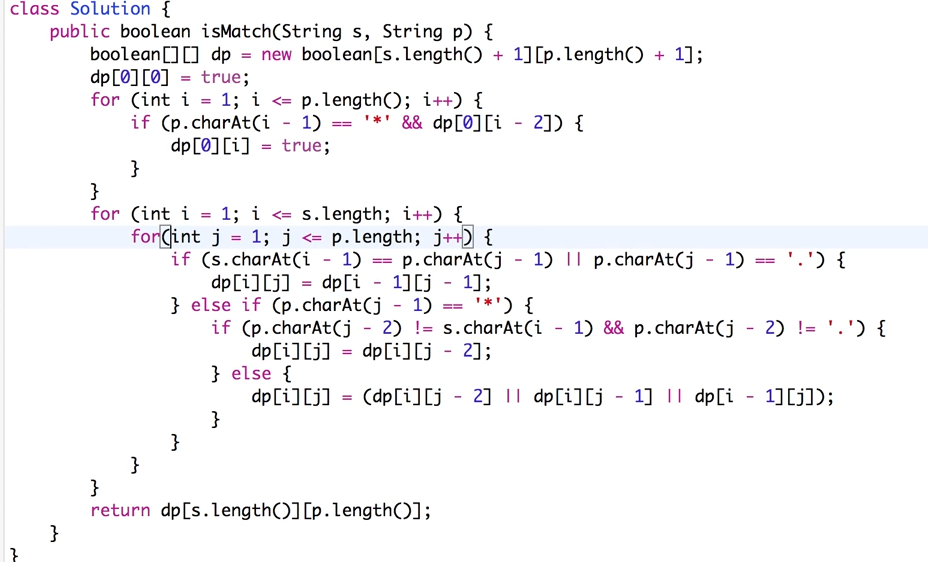base case: 0, 0, and if the s is empty and if p is ‘.*’ or ‘c*’, then it is true.

241. Different Ways to Add Parentheses

Given a string of numbers and operators, return all possible results from computing all the different possible ways to group numbers and operators. The valid operators are `+`, `-` and `*`.

Example 1

Input: `"2-1-1"`.

`((2-1)-1) = 0(2-(1-1)) = 2`

Output: `[0, 2]`

Example 2

Input: `"2*3-4*5"`

`(2*(3-(4*5))) = -34((2*3)-(4*5)) = -14((2*(3-4))*5) = -10(2*((3-4)*5)) = -10(((2*3)-4)*5) = 10`

Output: `[-34, -14, -10, -10, 10]`

Solution: to add a parenthese is the same as splitting there. T(n)=T(1)+T(n-1)+T(2)+T(n-2)+…+: T(n)=T(0,i-1)optT(i+1,n) (i=[1,n])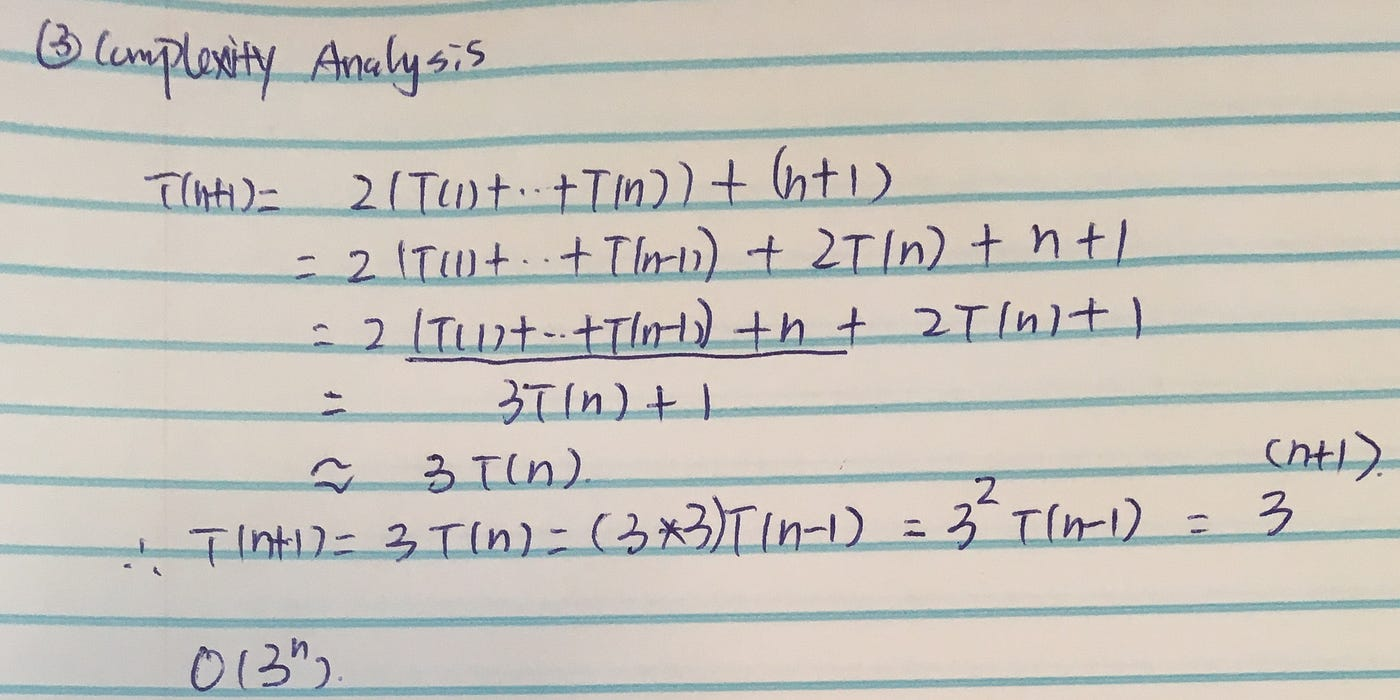Deduct the complexity for the non-standard problems, more might use the Catalan_number

Code:

`def diffWaysToCompute(self, input):        """        :type input: str        :rtype: List[int]        """        if not input:            return []        #convert it to list        ops={'*','-','+'}        nums =[]        substring = ''        for c in input:            if c in ops:                nums.append(int(substring))                nums.append(c)                                substring=''            else:                substring+=c        nums.append(int(substring))                def operator(lst1,lst2,op):            rslt=[]            for x in lst1:                for y in lst2:                    if op=='*':                        rslt.append(x*y)                    elif op=='+':                        rslt.append(x+y)                    else:                        rslt.append(x-y)                                return rslt                                               def split(low,high):            if low==high:                return [nums[low]]            rslt=[]            for i in xrange(low+1,high,2):                rslt+=operator(split(low,i-1),split(i+1,high),nums[i])            return rslt                return split(0,len(nums)-1)`

With memorization:

`memo = [[None for col in xrange(len(nums))] for row in xrange(len(nums))]                     def split(low,high):            if low==high:                return [nums[low]]            #check memo            if memo[low][high]:                return memo[low][high]            rslt=[]            for i in xrange(low+1,high,2):                rlst_left, rslt_right = [],[]                #check memo                if memo[low][i-1]:                    rslt_left=memo[low][i-1]                else:                    rslt_left = split(low,i-1)                if memo[i+1][high]:                    rslt_right=memo[i+1][high]                else:                    rslt_right = split(i+1,high)                rslt+=operator(rslt_left,rslt_right,nums[i])            #save memo            memo[low][high] = rslt            return rslt                return split(0,len(nums)-1)`

Code optimization

`tokens = re.split('(\D)', input)   nums = map(int, tokens[::2])   ops = map({'+': operator.add, '-': operator.sub, '*': operator.mul}.get, tokens[1::2])`

10. Regular Expression Matching

Implement regular expression matching with support for `'.'` and `'*'`.

`'.' Matches any single character.'*' Matches zero or more of the preceding element.The matching should cover the entire input string (not partial).The function prototype should be:bool isMatch(const char *s, const char *p)Some examples:isMatch("aa","a") → falseisMatch("aa","aa") → trueisMatch("aaa","aa") → falseisMatch("aa", "a*") → trueisMatch("aa", ".*") → trueisMatch("ab", ".*") → trueisMatch("aab", "c*a*b") → true`

Solution: it is important to understand that if .* means we can match any preceding elements from len 0 to any. c* means we can match zero or more c. My solution is to reverse the string, and with memorization, the running became half of it.

`def isMatch(self, s, p):        """        :type s: str        :type p: str        :rtype: bool        """        memo=[[-1 for col in range(len(p)+1)] for row in range(len(s)+1)]        s, p=s[::-1], p[::-1]                def match(i,j):            if memo[i][j]!=-1:                return memo[i][j]            if i<len(s) and j==len(p): #string left #if pattern left                memo[i][j]=0                return False            if i==len(s) and j<len(p):                if p[j] is not '*': #if pattern left and is not '*', it is false                    memo[i][j]=0                    return False            if i==len(s) and j==len(p):                memo[i][j]=1                return True            if p[j]=='.':                memo[i][j] = 1 if match(i+1, j+1) else 0                return memo[i][j]==1            elif p[j]=='*':                if p[j+1]=='.':                    if j+2==len(p):                        return True                    #if there are charaters left, e.g."aab" "b.*", we need to do another match                    if any(match(k+i,j+2) for k in range(len(s)-i+1)):                        memo[i][j]=1                        return True                    memo[i][j]=0                    return False                if match(i, j+2): #match ''                    memo[i][j]=1                    return True                for k in range(0,len(s)-i):                                       if p[j+1]==s[k+i]: #match 1 or more                        if match(k+i+1, j+2):                            memo[i][j]=1                            return True                    else:                        break                memo[i][j]=0return False            else:                if s[i]==p[j]:                    memo[i][j]=1 if match(i+1,j+1) else 0                    return memo[i][j]==1                else:                    memo[i][j] =0                    return False        return match(0,0)`

Or use DP:

139. Word Break

Given a non-empty string s and a dictionary wordDict containing a list of non-empty words, determine if s can be segmented into a space-separated sequence of one or more dictionary words. You may assume the dictionary does not contain duplicate words.

For example, given
s = `"leetcode"`,
dict = `["leet", "code"]`.

Return true because `"leetcode"` can be segmented as `"leet code"`.

Solution: T(n) = T(n-1)+T(n-2)+…+T(1)+O(n). Without memoriazation, it is O(2^n). With it, then the time complexity is the same as the subproblems, which means the size of the memo. O(n²).

`def wordBreak(self, s, wordDict):        """        :type s: str        :type wordDict: List[str]        :rtype: bool        """        memo =[[None for col in range(len(s))] for row in range(len(s))]        def dp(b,e): #i is the start, j is the end            if b==len(s):                return False            if memo[b][e-1] is None:                if s[b:e] in wordDict:                    memo[b][e-1]=True                    return True                memo[b][e-1] =any( dp(b+i,e) and s[b:b+i] in wordDict for i in range(1,len(s)-b))            return memo[b][e-1]        return dp(0,len(s))`

140. Word Break II

Given a non-empty string s and a dictionary wordDict containing a list of non-empty words, add spaces in s to construct a sentence where each word is a valid dictionary word. You may assume the dictionary does not contain duplicate words.

Return all such possible sentences.

For example, given
s = `"catsanddog"`,
dict = `["cat", "cats", "and", "sand", "dog"]`.

A solution is `["cats and dog", "cat sand dog"]`.

Solution: the difference compared with the first one is we need to find all possible solutions, compared with (any). And we need to record the results. split into ‘cat’+dp(sanddog), because the return of dp could be [‘sand dog’, ‘s and dog’ ] if s is in dictionary, so that we need to connect the result and return.

`def wordBreak(self, s, wordDict):        """        :type s: str        :type wordDict: List[str]        :rtype: List[str]        """                memo =[[None for col in range(len(s))] for row in range(len(s))]        def dp(b,e): #i is the start, j is the end            if b==len(s):                return ''            r=[]            if memo[b][e-1] is None:                if s[b:e] in wordDict: #cant return here, we need to find all the solutions                    r.append(s[b:e]) #['catsanddog']                #split into left and right, it is parallel as the result before                for i in range(1,len(s)-b):                    if s[b:b+i] in wordDict:                        right=dp(b+i,e)                        if right:                            for item in right: #might have multiple                                tmp = s[b:b+i]+' '+item                                r.append(tmp)                memo[b][e-1]=r            return memo[b][e-1]              return dp(0,len(s))`

# 6 Time Complexity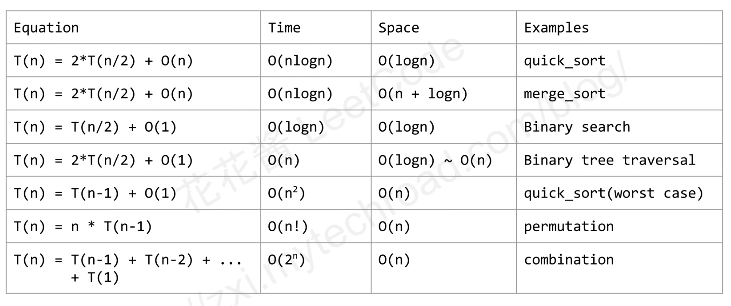This image shows the time complexity of divide and conquer and backtracking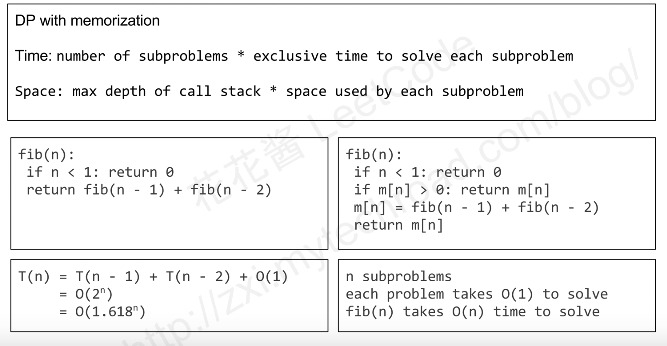This shows dynamic programming with or without memo, which convert an exponential time solution into a polynomial-time solution.

The space complexity is the height of the tree

## Time complexity theory from Introduction to Algorithms

For standard problems

substitution method

recursion-tree method

In a recursion tree, each node represents the cost of a single subproblem somewhere in the set of resursive function invocations. We sum the costs within each level of the tree to obtain a set of per-level costs, and then we sum all the per-level costs to determine the total cost of all levels of the recursion.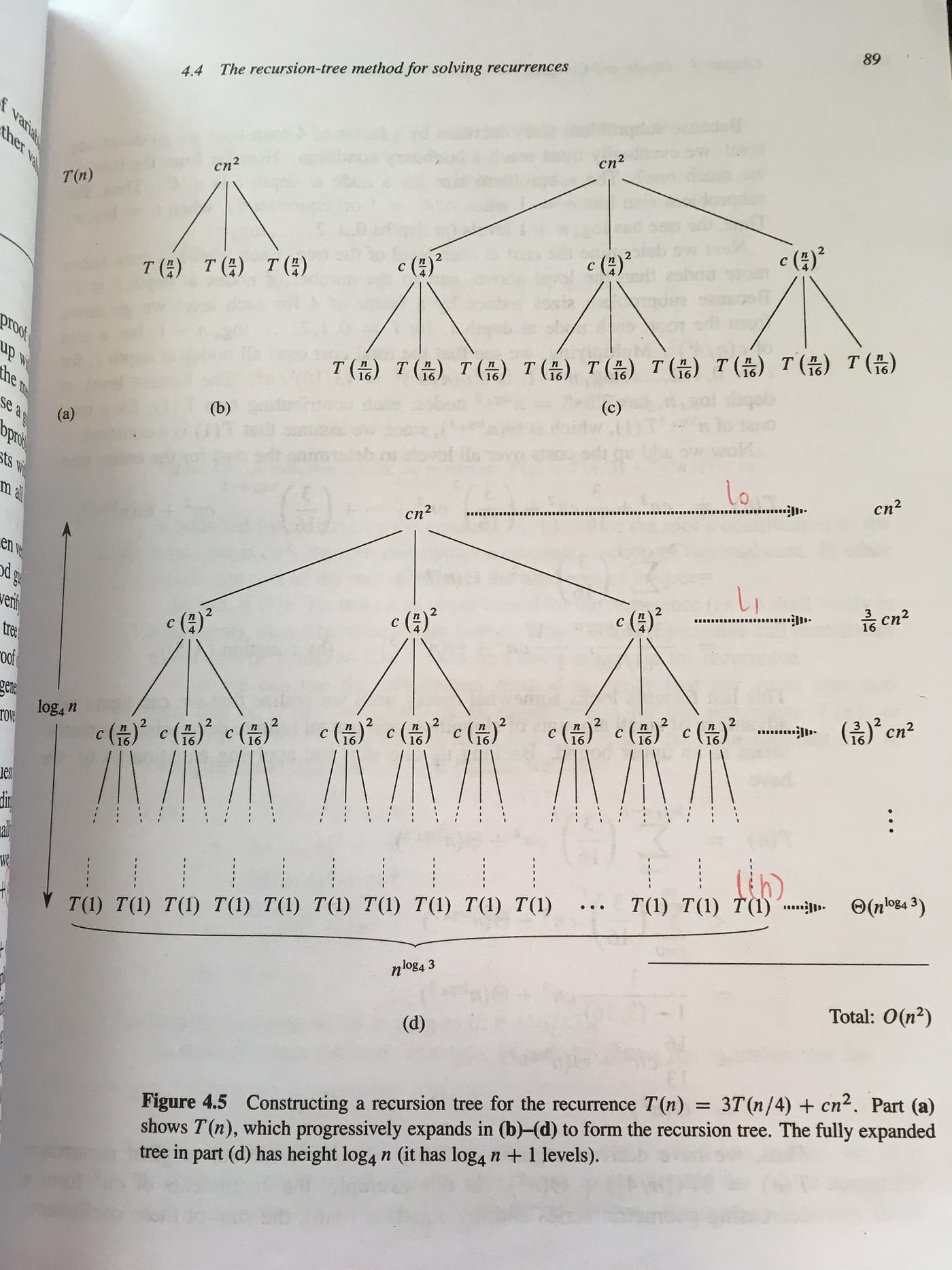For this equation, each time we divide the T(n) problem into subproblems, the cost is cn². from root to the last level(not the leaves), it is all divide operation cost. At the leaves, it represents the cost to really solve all of its final subprolems O(n^h).

master method

The master method provides a “cookbook” method for solving recurrences of the form:

T(n) =aT(n/b)+f(n)

where a≥1 and b>1 are constants and f(n) is an asymptotically positive function. It describes the running time of an algorithms that divides a problem of size n into a subprolems, each of since n/b

--

--